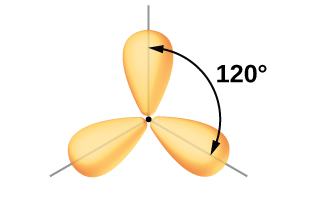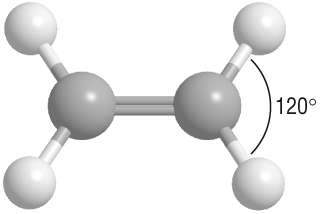Question #75709

Dec 11, 2016

The electron geometry about each carbon atom is b trigonal planar.

Explanation:

The molecule is symmetrical, so each carbon atom has the same electron domain geometry.

The left-hand carbon atom has three electron domains: the C=C double bond and the two C-C single bonds.Per VSEPR theory, three electron domains will point towards the corners of an equilateral triangle, with all angles equal to 120°.

Here's a ball-and-stick model that shows the electron domain geometry in your compound.